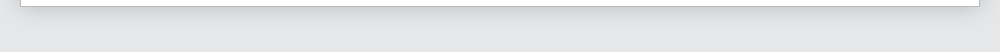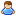Login Products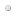SalesSupportDownloadsAbout
 Home » Technical Support » Elevate Web Builder Technical Support » Support Forums » Elevate Web Builder General » View Thread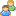View Thread

The following is the text of the current message along with any replies.
 Messages 1 to 4 of 4 total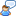Tedit Char filtering
 Sat, May 3 2014 3:13 PM Permanent Link E.B Hi, I'm trying to make a TEdit only accept numeric char (0,1,...,9). Using KeyPress or KeyDown event, trying to inspect char code to allow or not it didn't work for me ... "&" code is 49, like "1" one's ... So I'm not easily able to distinguish between them (and EWB does not propose "in [0..9]" instruction). Does someone knows a simple way to do that ? (I have the same problem with a TEdit that only accept [a..b,A..B] ). Eric. Sat, May 3 2014 4:30 PM Permanent Link Raul Globestar Systems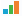Team Elevate On 5/3/2014 3:13 PM, E.B wrote: > I'm trying to make a TEdit only accept numeric char (0,1,...,9). > Using KeyPress or KeyDown event, trying to inspect char code > to allow or not it didn't work for me ... What did you try ? > Does someone knows a simple way to do that ? There are couple of ways. The simplest would be something like this in the edits Keypress :    if (Key >='0') AND (Key <= '9') then       result := true    else       result := false; However you'll notice that some letters can still be entered (since KeyPress is now always called) so better solution would be to to drop down to KeyDown event. The only problem here is that you likely want to handle some control codes still (like backspace for example) so try something like this in edit contrrols KeyDown event:    result := false;    if not (shiftkey) then    begin       if ( Key >= ord('0') ) AND ( key <= ord('9')) OR (Key=8) OR (Key=46) then result:= true;    end; Raul Sun, May 4 2014 4:12 PM Permanent Link E.B Raul wrote: On 5/3/2014 3:13 PM, E.B wrote: > I'm trying to make a TEdit only accept numeric char (0,1,...,9). > Using KeyPress or KeyDown event, trying to inspect char code > to allow or not it didn't work for me ... What did you try ? > Does someone knows a simple way to do that ? There are couple of ways. The simplest would be something like this in the edits Keypress :    if (Key >='0') AND (Key <= '9') then       result := true    else       result := false; However you'll notice that some letters can still be entered (since KeyPress is now always called) so better solution would be to to drop down to KeyDown event. The only problem here is that you likely want to handle some control codes still (like backspace for example) so try something like this in edit contrrols KeyDown event:    result := false;    if not (shiftkey) then    begin       if ( Key >= ord('0') ) AND ( key <= ord('9')) OR (Key=8) OR (Key=46) then result:= true;    end; Raul, the problem doesn't seems to be as simple as that ... Using my KeyBoard, key=49 when I press [SHIFT "&"] or "1" alone ... When I press [SHIFT "&"] the char wrote in Tedit is "1" When I press ["1"] alone the char wrote in Tedit is "&" In the two case, key=49. Eric. Sun, May 4 2014 4:39 PM Permanent Link E.B E.B wrote: Raul wrote: On 5/3/2014 3:13 PM, E.B wrote: > I'm trying to make a TEdit only accept numeric char (0,1,...,9). > Using KeyPress or KeyDown event, trying to inspect char code > to allow or not it didn't work for me ... What did you try ? > Does someone knows a simple way to do that ? There are couple of ways. The simplest would be something like this in the edits Keypress :    if (Key >='0') AND (Key <= '9') then       result := true    else       result := false; However you'll notice that some letters can still be entered (since KeyPress is now always called) so better solution would be to to drop down to KeyDown event. The only problem here is that you likely want to handle some control codes still (like backspace for example) so try something like this in edit contrrols KeyDown event:    result := false;    if not (shiftkey) then    begin       if ( Key >= ord('0') ) AND ( key <= ord('9')) OR (Key=8) OR (Key=46) then result:= true;    end; Raul, the problem doesn't seems to be as simple as that ... Using my KeyBoard, key=49 when I press [SHIFT "&"] or "1" alone ... When I press [SHIFT "&"] the char wrote in Tedit is "1" When I press ["1"] alone the char wrote in Tedit is "&" In the two case, key=49. Eric. Sorry Raul I'm a little bit stupid sometime ... SHIFT key could be use to choose between the two characters, ok. So I wrote that using your idea (it includes numpad): if (shiftkey) then begin    if ( key >= ord('0') ) AND ( key <= ord('9')) then    result:= true; end else begin   if (( key >= 96 ) AND ( key <= 105))      OR (Key=8) OR (Key=46) then    result:= true; end;